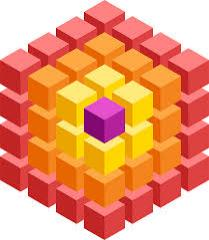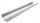# Cube surface area

The surface of the cube was originally 216 centimeters square. The surface of the cube has shrunk from 216 to 54 centimeters sq. Calculate how much percent the edge of the cube has decreased.

Correct result:

p =  50 %

#### Solution:We would be pleased if you find an error in the word problem, spelling mistakes, or inaccuracies and send it to us. Thank you!Tips to related online calculators

## Next similar math problems:

• Cardboard boxWe want to make a cardboard box shaped quadrangular prism with rhombic base. Rhombus has a side of 5 cm and 8 cm one diagonal long. The height of the box to be 12 cm. The box will be open at the top. How many square centimeters cardboard we need, if we ca
• Roof coverAbove the pavilion with a square ground plan with a side length of a = 12 m is a pyramid-shaped roof with a height v = 4.5 m. Calculate how much m2 of sheet metal is needed to cover this roof if 5.5% of the sheet we must add for joints and waste.
• PrismRight angle prism, whose base is right triangle with leg a = 3 cm and hypotenuse c = 13 cm has same volume as a cube with an edge length of 3 dm. a) Determine the height of the prism b) Calculate the surface of the prism c) What percentage of the cube's s
• Cube zoomHow many percents do we increase the volume and surface of the cube if we magnify its edge by 38 %?
• Content area and percentsDetermine what percentage is smaller cube surface, when the surface area of the wall decreases by 25%.
• PaintingThe room is long 50 meters and wide 60dm and 300 cm high. Calculate how much it will cost painting if area of windows and doors is 15% of the total area. One square meter cost 50cents.How many square meters is needed to cover the tower the shape of regular quadrangular pyramid base edge 10 meters, if the deviation lateral edges from the base plane is 68 °? Calculate coverage waste 10%.
• Surface area 2Calculate how many % reduce the surface area of the cube is we reduced length of each edge by 10%.
• Roof 7The roof has the shape of a regular quadrangular pyramid with a base edge of 12 m and a height of 4 m. How many percent is folds and waste if in construction was consumed 181.4m2 of plate?
• The tentThe tent shape of a regular quadrilateral pyramid has a base edge length a = 2 m and a height v = 1.8 m. How many m2 of cloth we need to make the tent if we have to add 7% of the seams? How many m3 of air will be in the tent?
• Cube into sphereThe cube has brushed a sphere as large as possible. Determine how much percent was the waste.
• The ballThe ball has a radius of 2m. What percentage of the surface and volume is another sphere whose radius is 20% larger?
• Metal sheetsHow much metal sheet is needed to produce 8 gutters 4 m long and 12 cm in diameter? During production joints calculate at 3% of total consumption.
• TowerThe top of the tower is a regular hexagonal pyramid with base edge 8 meters long and a height 5 meters. How many m2 of the sheet is required to cover the top of the tower if we count 8% of the sheet waste?
• Church roof 2The roof has the shape of a rotating cone shell with a base diameter of 6 m and a height of 2.5 m. How many monez (CZK) will cost the roof cover sheet if 1 m2 of metal sheet costs 152 CZK and if you need 15% extra for joints, overlays and waste?
• Volume and body diagonalCalculate how much the volume and body diagonal of the cuboid decrease if we reduce each of its three edges a, b, c by 18%?
• The roomThe room has a cuboid shape with dimensions: length 50m and width 60dm and height 300cm. Calculate how much this room will cost paint (floor is not painted) if the window and door area is 15% of the total area and 1m2 cost 15 euro.